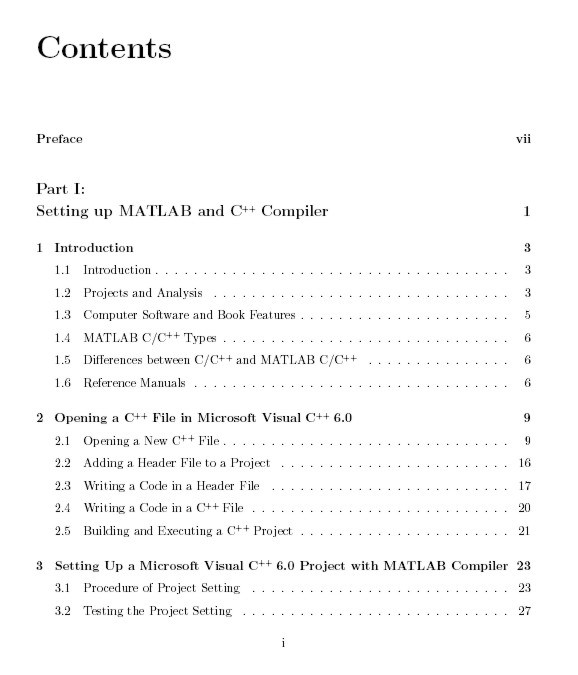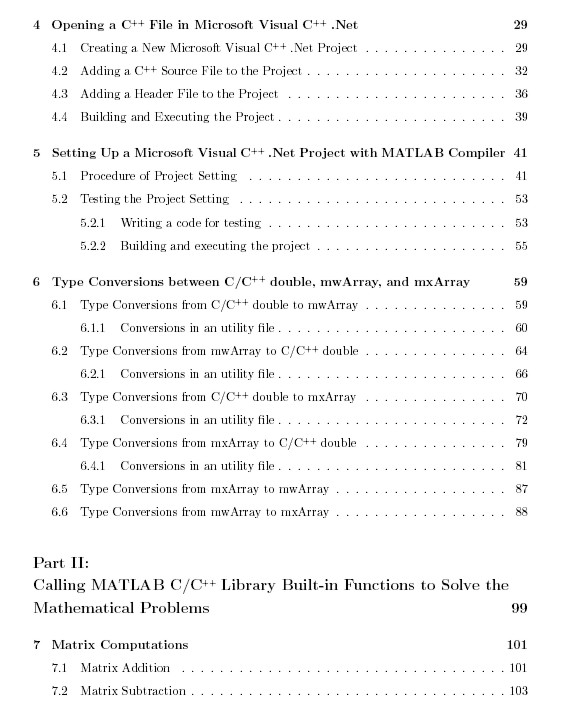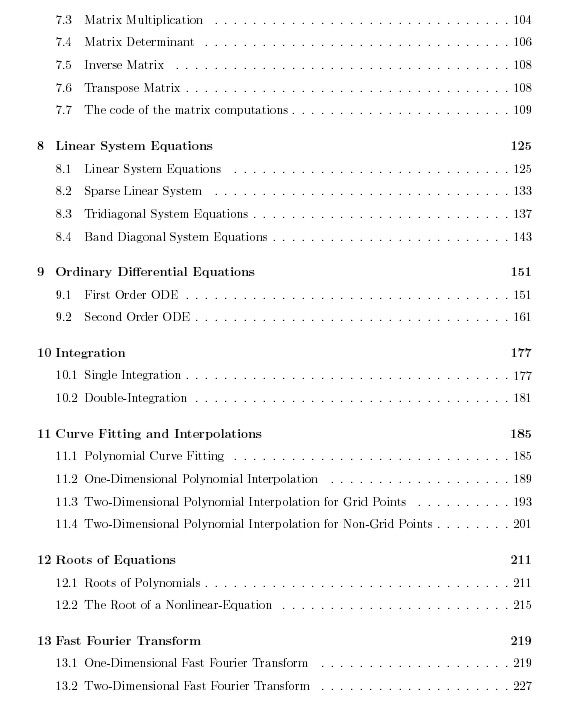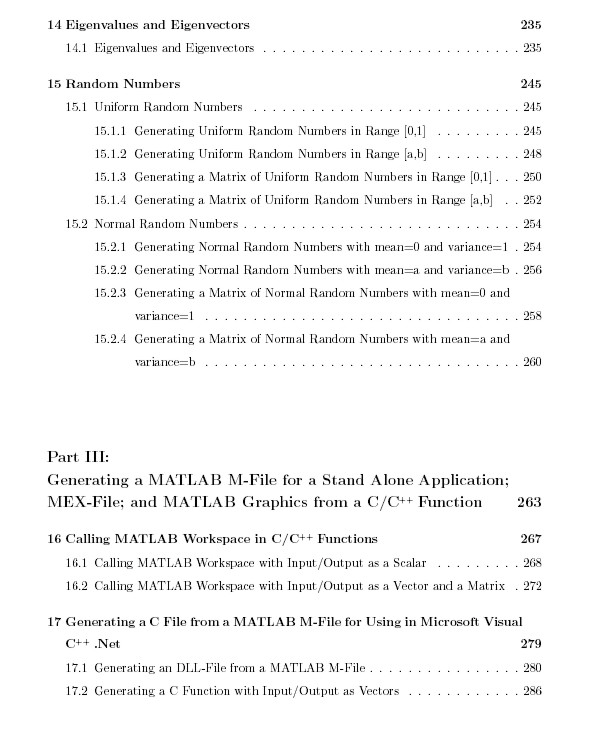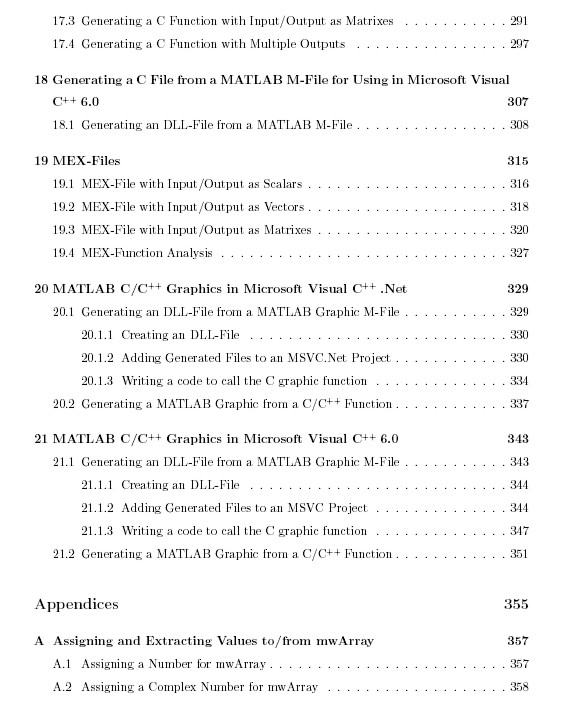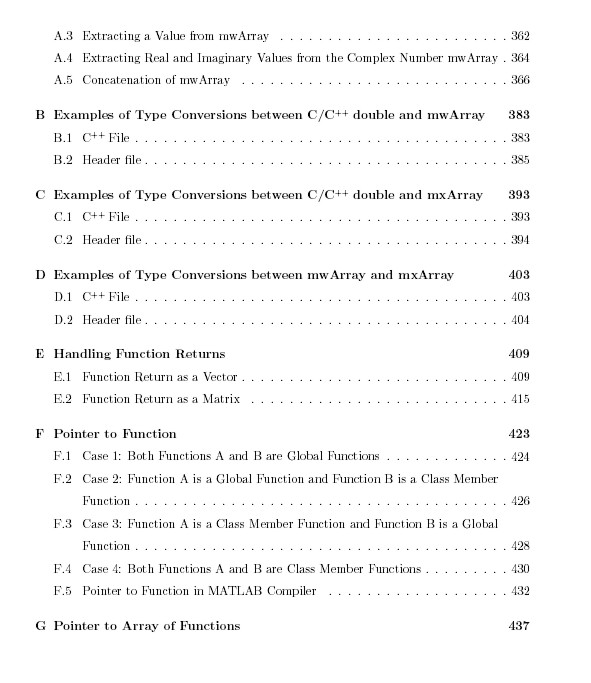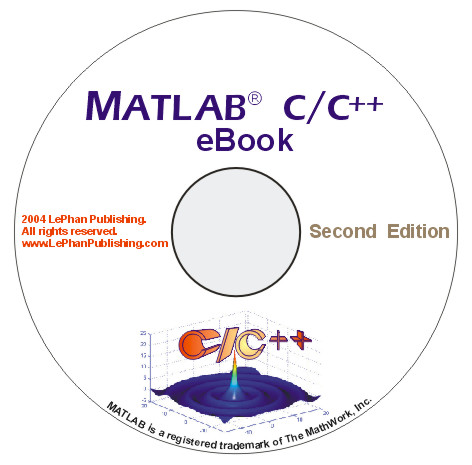# MATLAB®   C/C++  Book  for MATLAB Compiler 3.0 (ebook)

MATLAB C++ Book illustrates the works of the C/C++ programming and MATLAB. The features of this book are designed to handle the five following projects:

1. Working on C/C++ code, we call the mathematical built-in functions in MATLAB C/C++ Library to solve the mathematical problems.

2. Working on C/C++ code, we call the MATLAB workspace to perform a particular task in the MATLAB workspace then transfer back results to a C/C++ function.

3. From an existing M-function, we generate a C function in a dll file for a Stand Alone Application.

4. From an existing C/C++ function, we call this function in MATLAB by writing a MEX- function for this function.

5. Working on C/C++ code, we want to use MATLAB graphics.

In addition, the book has chapters to help you set up Microsoft Visual C++ 6.0 and Microsoft Visual C++ .Net with MATLAB Compiler 3.0.

The MATLAB C++ Book is in the pdf format, and also includes the complete C/C++ code examples of 21 chapters and 7 appendices. The example codes are developed, compiled, and tested in:

. MATLAB R13 (6.5) and MATLAB Compiler 3.0

This MATLAB C++ Book is a great support for C/C++ programmers who are using MATLAB to develop applications and solutions. The book is promoted at the websites MATLAB and Technical Book Resources.

Using the combination of both tools, C/C++ and MATLAB, you have the best tool in your hand to solve the technical problems.

MATLAB and MATLAB Compiler are registered trademarks of The MathWork, Inc.
Microsoft, Windows, and Microsoft Visual C++ are